### Skin Effects

 Skin EffectsIt is difficult for electromagnetic waves to penetrate into a conductor because of severe impedance matching. The impedance of a conductor is given by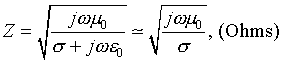where sigma (Siemens/m) is the conductivity. Noting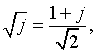we may write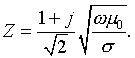Even in microwave frequency regime, this is much smaller than the impedance of free space (377Ohms) because of large value of conductivity of ordinary conductors. The imaginary part of theimpedance indicates strong dissipation of electromagnetic energy into heat.    Electromagnetic waves can penetrate into a conductor to a depth of order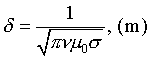This quantity is called the "skin depth." When a dc field is suddenly applied to a conductor, the filed will eventually fully penetrate but it takes about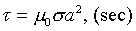for the field to do so. Here, a is a characteristic size of a conductor (e.g., thickness of slab, radius of sphere, etc.) This is called "skin time."  Animation at the top shows penetration of an oscillating field, and bottom animation shows transient penetration of a dc field into a slab.  with(plots):  animate(cos(2*Pi*.1*t)*Heaviside(-x)+Heaviside(x)*exp(-x)*cos(x-2*Pi*.1*t),x=-2..7,t=0..9,frames=20,color=red,numpoints=50);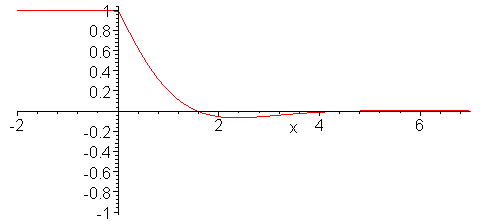with(plots):  animate(Heaviside(abs(x)-.5)+(1.-(4./Pi)*(cos(Pi*x)*exp(-t)-cos(3*Pi*x)*exp(-9*t)/3+cos(5*Pi*x)*exp(-25*t)/5-cos(7*Pi*x)*exp(-49*t)/7+cos(9*Pi*x)*exp(-81*t)/9-cos(11*Pi*x)*exp(-121*t)/11+cos(13*Pi*x)*exp(-169*t)/13))*Heaviside(.5-abs(x)),x=-2..2,t=0.01..10,frames=50,color=red,numpoints=100);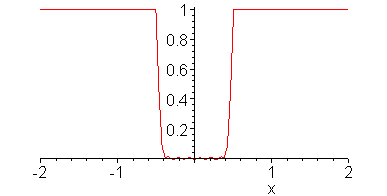Ċ
Jason Lin,
2018年9月6日 凌晨12:42